# AP Physics C: Mechanics : Interpreting Circular Motion Diagrams

## Example Questions

### Example Question #1 : Circular And Rotational Motion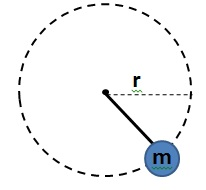A ball of massis tied to a rope and moves along a horizontal circular path of radiusas shown in the diagram (view from above). The maximum tension the rope can stand before breaking is given by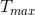. Which of the following represents the ball's linear velocity given that the rope does not break?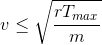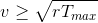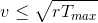None of these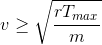Explanation:

This is a centripetal force problem. In this case the tension on the rope is the centripetal force that keeps the ball moving on a circle.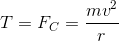If we want for the rope not to break, then the tension should never exceed.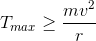Now we just solve for velocity: